# Math - Derivative (Sensitivity to Change, Differentiation)

The derivative of a function of a real variable measures the sensitivity to change of a quantity (a function or dependent variable) which is determined by another quantity (the independent variable).

What will happens to Y if I change X a little bit ? $$f(X) = Y$$ where:

The derivative measures the instantaneous rate of change of the function, as distinct from its average rate of change.

The derivative of the position of a moving object with respect to time is the object's velocity. This measures how quickly the position of the object changes when time is advanced.

## Example

Made with the Math.js library that implements this wiki/Differentiation rules for computing the derivative of a function in calculus.

console.log('calculate derivatives');
console.log(math.derivative('2x^2 + 3x + 4', 'x').toString());  // '4 * x + 3'
console.log(math.derivative('sin(2x)', 'x').toString());        // '2 * cos(2 * x)'


## Formula

The derivative of a function at a chosen input value describes the best linear approximation of the function near that input value.

In fact, the derivative at a point of a function of a single variable is:

• the slope
• of the tangent line

to the graph of the function at that point.

## Documentation / Reference

Recommended Pages(Stream|Pipe|Message Queue|Event Processing)

From an abstract point of view, a stream is a sequence of aninfinite cardinality (size) delivered at unknown time intervals. list Streams: are inputs and outputs of operations may be also buffers...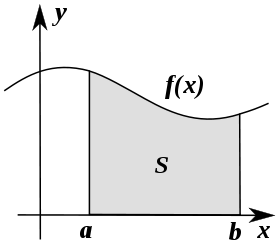Calculus - Integral

Integration can be thought of as measuring the area under a curve, defined by f(x), between two points (here a and b). Riemann integral was the first rigorous definition of the integral of a function...Rate of Change

the functions usually represent physical quantities, the derivatives represent their rates of change, and the equation defines a relationship between the two. Differential_equation The derivative...Statistic - Smooth (Function Continuity) (Soft ?)

To smooth a data set is to create an approximating function that attempts to capture important patterns in the data, while leaving out noise. In mathematical analysis, smoothness has to do with how many...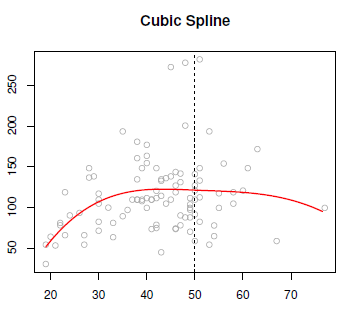Statistics - Cubic spline

Cubic splines is a step cubic piecewise polynomial in the left and the right and continuous at the knot. There's the following constraints: The first derivatives continues at the knot, and the...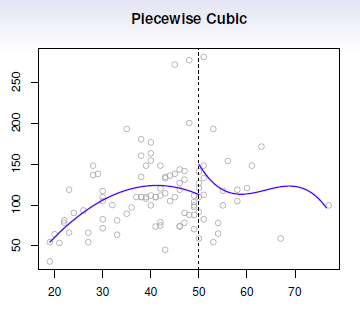Statistics - Piecewise polynomials

Piecewise polynomials generalize the idea of piecewise constants. The idea try to get rid of the global polynomial because it's global and not local. Instead of having asingle polynomial over the whole...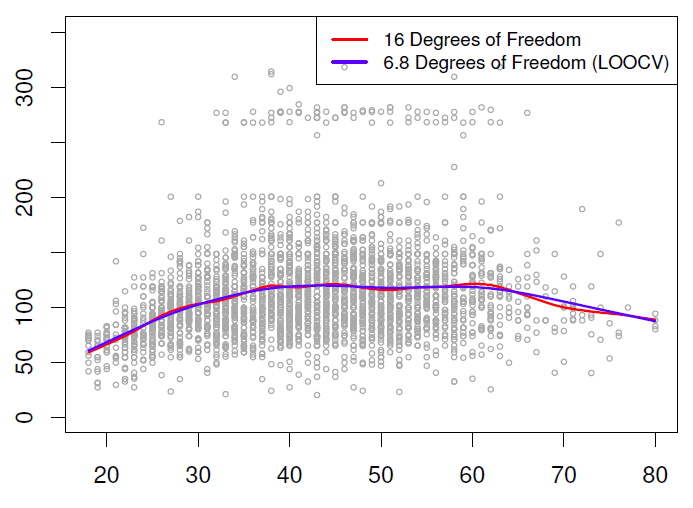Statistics - Smoothing (cubic) spline

A smoothing spline is a way of fitting splines without having to worry knots. They are a little bit more challenging mathematically as others splines and approaches the problem from a completely different...Time - Moment (Time derivative)

A moment can be seen as the derivative of a variable against time. A variable may evolve in time while being random at each particular moment.Trigonometry - (Cosine|Cosinus)

Cosine is the function of an angle (trigonometric function) For the cosine function: The derivative is the sine: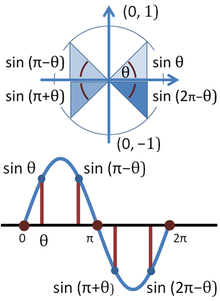Trigonometry - (Sine|Sinus)

Sine is the function of an angle (trigonometric function). The word comes from the Latin sinus for gulf or bay, since, given a unit circle, it is the side of the triangle on which the angle opens. ...